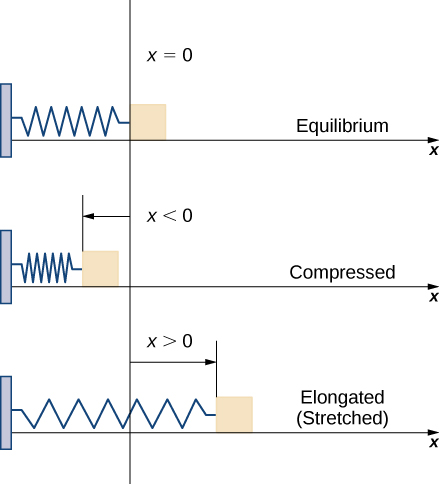# 6.5 Physical applications  (Page 3/11)

 Page 3 / 11
${W}_{i}\approx F\left({x}_{i}^{*}\right)\left({x}_{i}-{x}_{i-1}\right)=F\left({x}_{i}^{*}\right)\text{Δ}x.$

Therefore, the work done over the interval $\left[a,b\right]$ is approximately

$W=\sum _{i=1}^{n}{W}_{i}\approx \sum _{i=1}^{n}F\left({x}_{i}^{*}\right)\text{Δ}x.$

Taking the limit of this expression as $n\to \infty$ gives us the exact value for work:

$W=\underset{n\to \infty }{\text{lim}}\sum _{i=1}^{n}F\left({x}_{i}^{*}\right)\text{Δ}x={\int }_{a}^{b}F\left(x\right)dx.$

Thus, we can define work as follows.

## Definition

If a variable force $F\left(x\right)$ moves an object in a positive direction along the x -axis from point a to point b , then the work    done on the object is

$W={\int }_{a}^{b}F\left(x\right)dx.$

Note that if F is constant, the integral evaluates to $F·\left(b-a\right)=F·d,$ which is the formula we stated at the beginning of this section.

Now let’s look at the specific example of the work done to compress or elongate a spring. Consider a block attached to a horizontal spring. The block moves back and forth as the spring stretches and compresses. Although in the real world we would have to account for the force of friction between the block and the surface on which it is resting, we ignore friction here and assume the block is resting on a frictionless surface. When the spring is at its natural length (at rest), the system is said to be at equilibrium. In this state, the spring is neither elongated nor compressed, and in this equilibrium position the block does not move until some force is introduced. We orient the system such that $x=0$ corresponds to the equilibrium position (see the following figure).A block attached to a horizontal spring at equilibrium, compressed, and elongated.

According to Hooke’s law    , the force required to compress or stretch a spring from an equilibrium position is given by $F\left(x\right)=kx,$ for some constant $k.$ The value of $k$ depends on the physical characteristics of the spring. The constant $k$ is called the spring constant and is always positive. We can use this information to calculate the work done to compress or elongate a spring, as shown in the following example.

## The work required to stretch or compress a spring

Suppose it takes a force of $10$ N (in the negative direction) to compress a spring $0.2$ m from the equilibrium position. How much work is done to stretch the spring $0.5$ m from the equilibrium position?

First find the spring constant, $k.$ When $x=-0.2,$ we know $F\left(x\right)=-10,$ so

$\begin{array}{ccc}\hfill F\left(x\right)& =\hfill & kx\hfill \\ \hfill -10& =\hfill & k\left(-0.2\right)\hfill \\ \hfill k& =\hfill & 50\hfill \end{array}$

and $F\left(x\right)=50x.$ Then, to calculate work, we integrate the force function, obtaining

$W={\int }_{a}^{b}F\left(x\right)dx={\int }_{0}^{0.5}50x\phantom{\rule{0.2em}{0ex}}dx={25{x}^{2}|}_{0}^{0.5}=6.25.$

The work done to stretch the spring is $6.25$ J.

Suppose it takes a force of $8$ lb to stretch a spring $6$ in. from the equilibrium position. How much work is done to stretch the spring $1$ ft from the equilibrium position?

$8$ ft-lb

## Work done in pumping

Consider the work done to pump water (or some other liquid) out of a tank. Pumping problems are a little more complicated than spring problems because many of the calculations depend on the shape and size of the tank. In addition, instead of being concerned about the work done to move a single mass, we are looking at the work done to move a volume of water, and it takes more work to move the water from the bottom of the tank than it does to move the water from the top of the tank.

We examine the process in the context of a cylindrical tank, then look at a couple of examples using tanks of different shapes. Assume a cylindrical tank of radius $4$ m and height $10$ m is filled to a depth of 8 m. How much work does it take to pump all the water over the top edge of the tank?

any genius online ? I need help!!
Pina
need to learn polynomial
Zakariya
i will teach...
nandu
I'm waiting
Zakariya
evaluate the following computation (x³-8/x-2)
teach me how to solve the first law of calculus.
what is differentiation
f(x) = x-2 g(x) = 3x + 5 fog(x)? f(x)/g(x)
fog(x)= f(g(x)) = x-2 = 3x+5-2 = 3x+3 f(x)/g(x)= x-2/3x+5
diron
pweding paturo nsa calculus?
jimmy
how to use fundamental theorem to solve exponential
find the bounded area of the parabola y^2=4x and y=16x
what is absolute value means?
Chicken nuggets
Hugh
🐔
MM
🐔🦃 nuggets
MM
(mathematics) For a complex number a+bi, the principal square root of the sum of the squares of its real and imaginary parts, √a2+b2 . Denoted by | |. The absolute value |x| of a real number x is √x2 , which is equal to x if x is non-negative, and −x if x is negative.
Ismael
find integration of loge x
find the volume of a solid about the y-axis, x=0, x=1, y=0, y=7+x^3
how does this work
Can calculus give the answers as same as other methods give in basic classes while solving the numericals?
log tan (x/4+x/2)
Rohan
Rohan
y=(x^2 + 3x).(eipix)
Claudia
Ismael
A Function F(X)=Sinx+cosx is odd or even?
neither
David
Neither
Lovuyiso
f(x)=1/1+x^2 |=[-3,1]
apa itu?
fauzi
determine the area of the region enclosed by x²+y=1,2x-y+4=0
Hi
MP
Hi too
Vic
hello please anyone with calculus PDF should share
Which kind of pdf do you want bro?
Aftab
hi
Abdul
can I get calculus in pdf
Abdul
explain for me
Usman
okay I have such documents
Fitzgerald
Hamza

#### Get Jobilize Job Search Mobile App in your pocket Now!By OpenStaxBy OpenStaxBy OpenStaxBy OpenStaxBy Jonathan LongBy OpenStaxBy OpenStaxBy Robert MurphyBy Tony PizurBy OpenStax